# 3.1: Simple example of kriging in gempy¶

In this notebook it will be shown how to create a kriged or simulated field in a simple geological model in gempy. We start by creating a simple model with three horizontally layered units, as shown in the gempy examples.

Importing GemPy

import gempy as gp

# Importing auxiliary libraries
import numpy as np
import matplotlib.pyplot as plt

# new for this
from gempy.assets import kriging

np.random.seed(5555)


Creating the model by importing the input data and displaying it:

data_path = 'https://raw.githubusercontent.com/cgre-aachen/gempy_data/master/'
geo_data = gp.create_data('kriging', extent=[0, 1000, 0, 50, 0, 1000], resolution=[50, 1, 50],
path_o=data_path + "/data/input_data/jan_models/model1_orientations.csv",
path_i=data_path + "/data/input_data/jan_models/model1_surface_points.csv")


Out:

Active grids: ['regular']


Setting and ordering the units and series:

gp.map_stack_to_surfaces(geo_data, {"Strat_Series": ('rock2', 'rock1'),
"Basement_Series": ('basement')})

surface series order_surfaces color id
0 rock2 Strat_Series 1 #015482 1
1 rock1 Strat_Series 2 #9f0052 2
2 basement Basement_Series 1 #ffbe00 3

Calculating the model:

interp_data = gp.set_interpolator(geo_data, compile_theano=True,
theano_optimizer='fast_compile')


Out:

Setting kriging parameters to their default values.
Compiling theano function...
Level of Optimization:  fast_compile
Device:  cpu
Precision:  float64
Number of faults:  0
Compilation Done!
Kriging values:
values
range              1415.1
$C_o$            47678.57
drift equations    [3, 3]


no mesh computed as basically 2D model

sol = gp.compute_model(geo_data, compute_mesh=False)


So here is the very simple, basically 2D model that we created:

gp.plot_2d(geo_data, cell_number=0, show_data=False)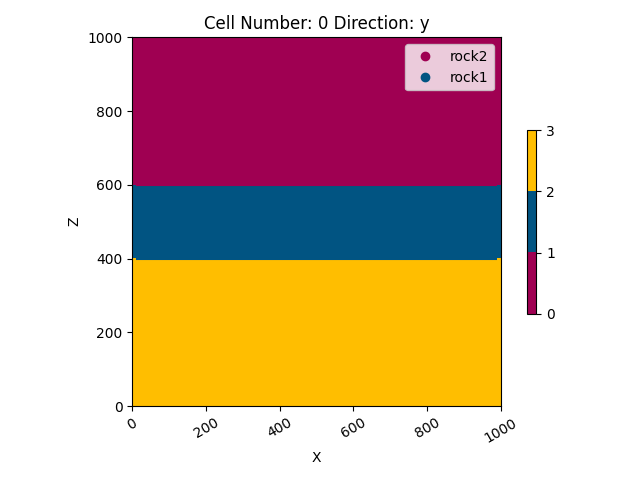Out:

<gempy.plot.visualization_2d.Plot2D object at 0x7fcc6baa02e0>


## 1) Creating domain¶

Let us assume we have a couple of measurements in a domain of interest within our model. In our case the unit of interest is the central rock layer (rock1). In the kriging module we can define the domain by handing over a number of surfaces by id - in this case the id of rock1 is 2. In addition we define four input data points in cond_data, each defined by x,y,z coordinate and a measurement value.

conditioning data (data measured at locations)

cond_data = np.array([[100, .5, 500, 2], [900, .5, 500, 1],
[500, .5, 550, 1], [300, .5, 400, 5]])


creating a domain object from the gempy solution, a defined domain conditioning data

domain = kriging.domain(model=sol, domain=, data=cond_data)


## 2) Creating a variogram model¶

variogram_model = kriging.variogram_model(theoretical_model='exponential',
range_=200, sill=np.var(cond_data[:, 3]))

variogram_model.plot(type_='both', show_parameters=True)
plt.show()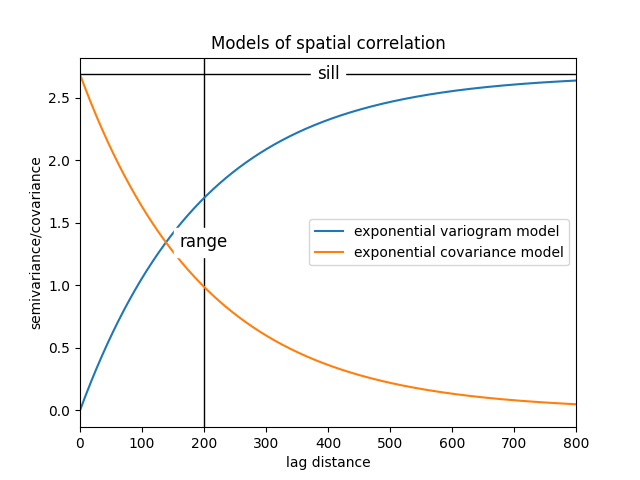## 3) Kriging interpolation¶

In the following we define an object called kriging_model and set all input parameters. Finally we generate the kriged field.

solution = kriging.create_kriged_field(domain, variogram_model)


The result of our calculation is saved in the following dataframe, containing an estimated value and the kriging variance for each point in the grid:

solution.results_df.head()

X Y Z estimated value estimation variance
0 10.0 25.0 410.0 2.24 2.13
1 10.0 25.0 430.0 2.20 2.01
2 10.0 25.0 450.0 2.15 1.90
3 10.0 25.0 470.0 2.10 1.81
4 10.0 25.0 490.0 2.07 1.77

It is also possible to plot the results in cross section similar to the way gempy models are plotted.

solution.plot_results(geo_data=geo_data, prop='val', contour=False,
direction='y', cell_number=0, alpha=0.7,
show_data=False, legend=True)
plt.show()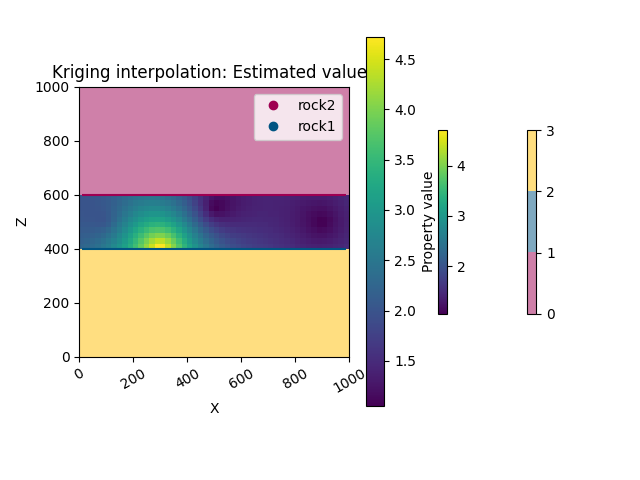Out:

/WorkSSD/PythonProjects/gempy/gempy/assets/kriging.py:265: MatplotlibDeprecationWarning: You are modifying the state of a globally registered colormap. In future versions, you will not be able to modify a registered colormap in-place. To remove this warning, you can make a copy of the colormap first. cmap = copy.copy(mpl.cm.get_cmap("viridis"))
cmap.set_bad(color='w', alpha=alpha) #define color and alpha for nan values

solution.plot_results(geo_data=geo_data, prop='both', contour=False,
direction='y', cell_number=0, alpha=0,
interpolation='bilinear', show_data=False)
plt.show()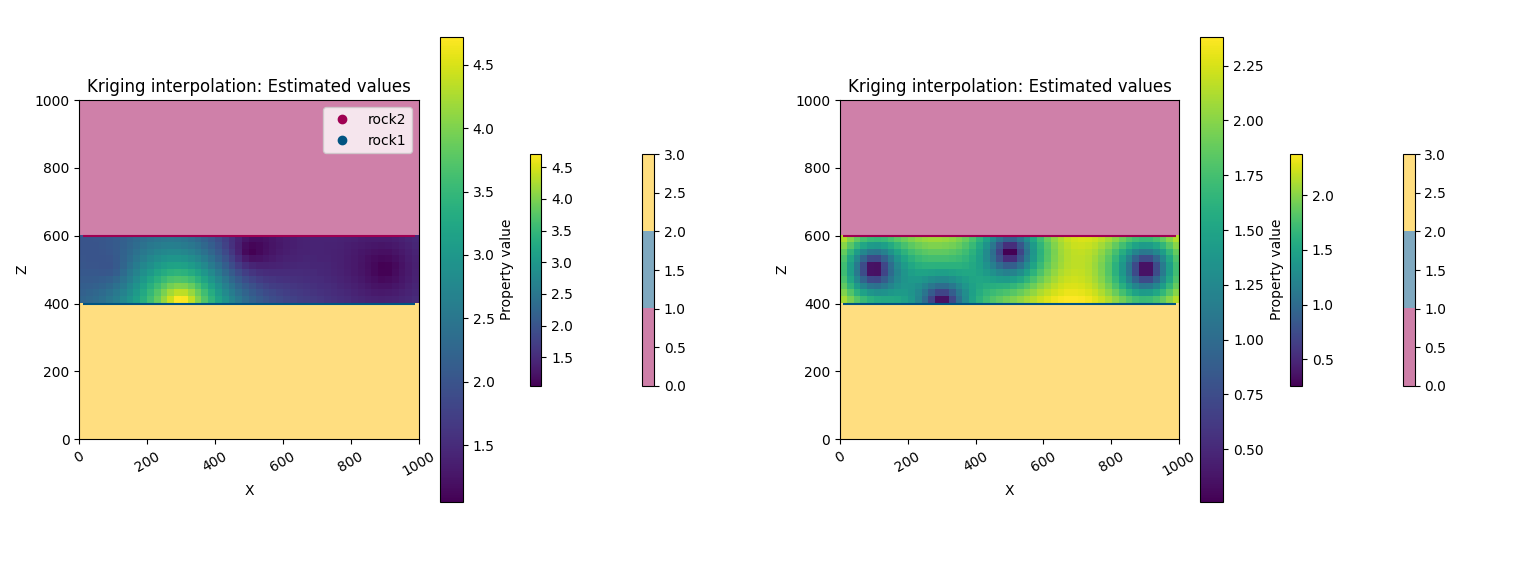Out:

/WorkSSD/PythonProjects/gempy/gempy/assets/kriging.py:265: MatplotlibDeprecationWarning: You are modifying the state of a globally registered colormap. In future versions, you will not be able to modify a registered colormap in-place. To remove this warning, you can make a copy of the colormap first. cmap = copy.copy(mpl.cm.get_cmap("viridis"))
cmap.set_bad(color='w', alpha=alpha) #define color and alpha for nan values


## 4) Simulated field¶

Based on the same objects (domain and varigoram model) also a simulated field (stationary Gaussian Field) can be generated. A Sequential Gaussian Simulation approach is applied in this module:

solution_sim = kriging.create_gaussian_field(domain, variogram_model)

solution_sim.results_df.head()

X Y Z estimated value estimation variance
16 10.0 25.0 410.0 2.61 1.48
157 10.0 25.0 430.0 2.38 0.28
276 10.0 25.0 450.0 3.98 0.24
25 10.0 25.0 470.0 2.54 0.53
267 10.0 25.0 490.0 0.76 0.28

solution_sim.results_df['estimated value']


Out:

16     2.61
157    2.38
276    3.98
25     2.54
267    0.76
...
55     3.76
165    3.32
427    2.01
303    1.96
187    2.04
Name: estimated value, Length: 500, dtype: float64


sphinx_gallery_thumbnail_number = 3

solution_sim.plot_results(geo_data=geo_data, prop='val', contour=False, direction='y', cell_number=0, alpha=0.7,
show_data=True, legend=True)
plt.show()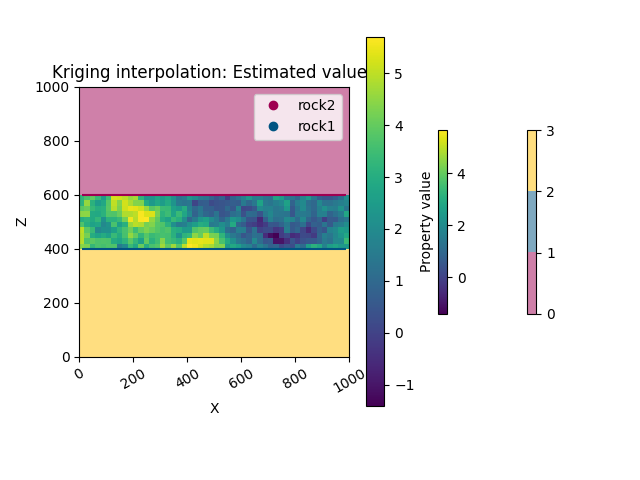Out:

/WorkSSD/PythonProjects/gempy/gempy/assets/kriging.py:265: MatplotlibDeprecationWarning: You are modifying the state of a globally registered colormap. In future versions, you will not be able to modify a registered colormap in-place. To remove this warning, you can make a copy of the colormap first. cmap = copy.copy(mpl.cm.get_cmap("viridis"))
cmap.set_bad(color='w', alpha=alpha) #define color and alpha for nan values

solution_sim.plot_results(geo_data=geo_data, prop='both', contour=False, direction='y', cell_number=0, alpha=0,
interpolation='bilinear', show_data=False)
plt.show()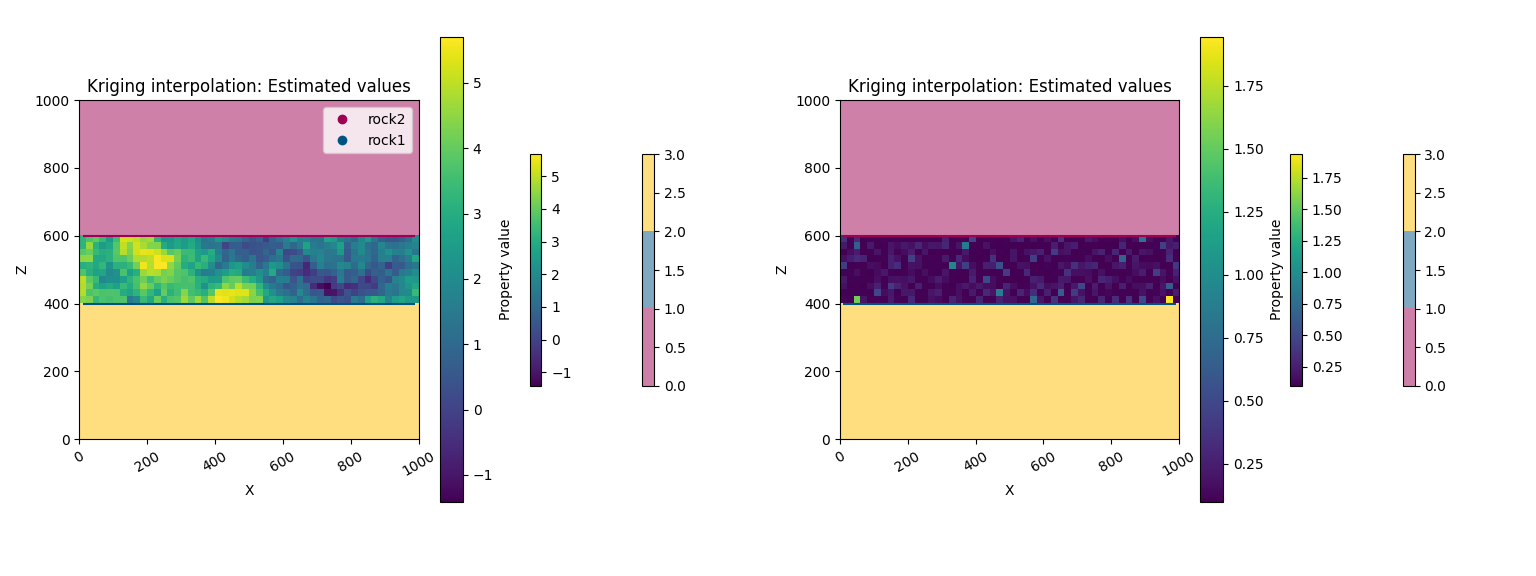Out:

/WorkSSD/PythonProjects/gempy/gempy/assets/kriging.py:265: MatplotlibDeprecationWarning: You are modifying the state of a globally registered colormap. In future versions, you will not be able to modify a registered colormap in-place. To remove this warning, you can make a copy of the colormap first. cmap = copy.copy(mpl.cm.get_cmap("viridis"))
cmap.set_bad(color='w', alpha=alpha) #define color and alpha for nan values


Total running time of the script: ( 0 minutes 7.495 seconds)

Gallery generated by Sphinx-Gallery